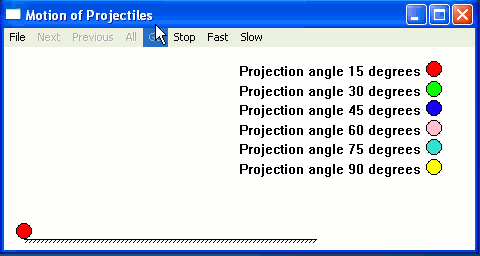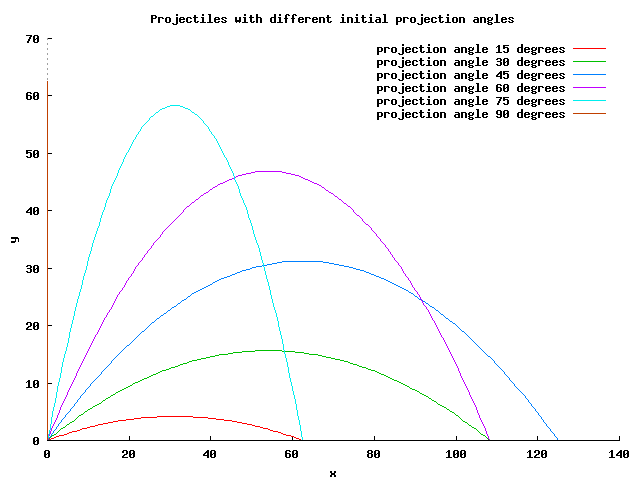Ch QuickAnimation Demos Motion of Projectiles Problem Statement: The same projectiles are fired into the air with the same initial speed 35.0 m/s, but with the different initial projection angles of 15 degrees, 45 degrees, 60 degrees, 75 degrees, and 90 degrees. Animate the motion of the projectiles and plot the traejctories of projectiles with 100 points for each trajectory. Animation This program projectiles.c uses Ch QuickAnimation to animate the motion of projectiles shown below. From the animation, we can clearly see that projectiles are landed at different time. The projectile with the initial projection angle of 15 degrees is landed the first. The projectile with the initial projection angle of 90 degrees is landed the last. The projectile with the initial projection angle of 45 degrees is landed the farthest. The projectiles with the initial projection angles of 15 degrees and 75 degrees are landed at the same place. The same case for the projectiles with the initial projection angles of 30 degrees and 60 degrees.Plotting The program projectiles.cpp uses Ch plotting capabilities to plot the traejctories of projectiles shown below